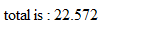# JavaScript: Sum of Decimal Array

 Tweet

Here’s how to do a sum of Array of Decimals in JavaScript. I have written this post to make you aware of the Array.prototype.reduce function, so that you do not have to impement one on your own.

Note: The reduce() method is a new method added to the Array object with JavaScript 1.8(ECMA 262- 5th ed). It is not supported by all browsers like IE8. Hence we will first useif (!Array.prototype.reduce) to check if the filter method is implemented in your browser, else provide an implementation for the reduce() method, so that it works on most browsers like IE8.

Here’s the reduce method as given in the Mozilla documentation

`if (!Array.prototype.reduce) {Array.prototype.reduce = function (fun /*, initialValue */) {    "use strict";    if (this === void 0  this === null)        throw new TypeError();    var t = Object(this);    var len = t.length >>> 0;    if (typeof fun !== "function")        throw new TypeError();    // no value to return if no initial value and an empty array    if (len == 0 && arguments.length == 1)        throw new TypeError();    var k = 0;    var accumulator;    if (arguments.length >= 2) {        accumulator = arguments;    }    else {        do {            if (k in t) {                accumulator = t[k++];                break;            }            // if array contains no values, no initial value to return            if (++k >= len)                throw new TypeError();        }        while (true);    }    while (k < len) {        if (k in t)            accumulator = fun.call(undefined, accumulator, t[k], k, t);        k++;    }    return accumulator;};}`

and we will implement this method as follows:

OUTPUT: